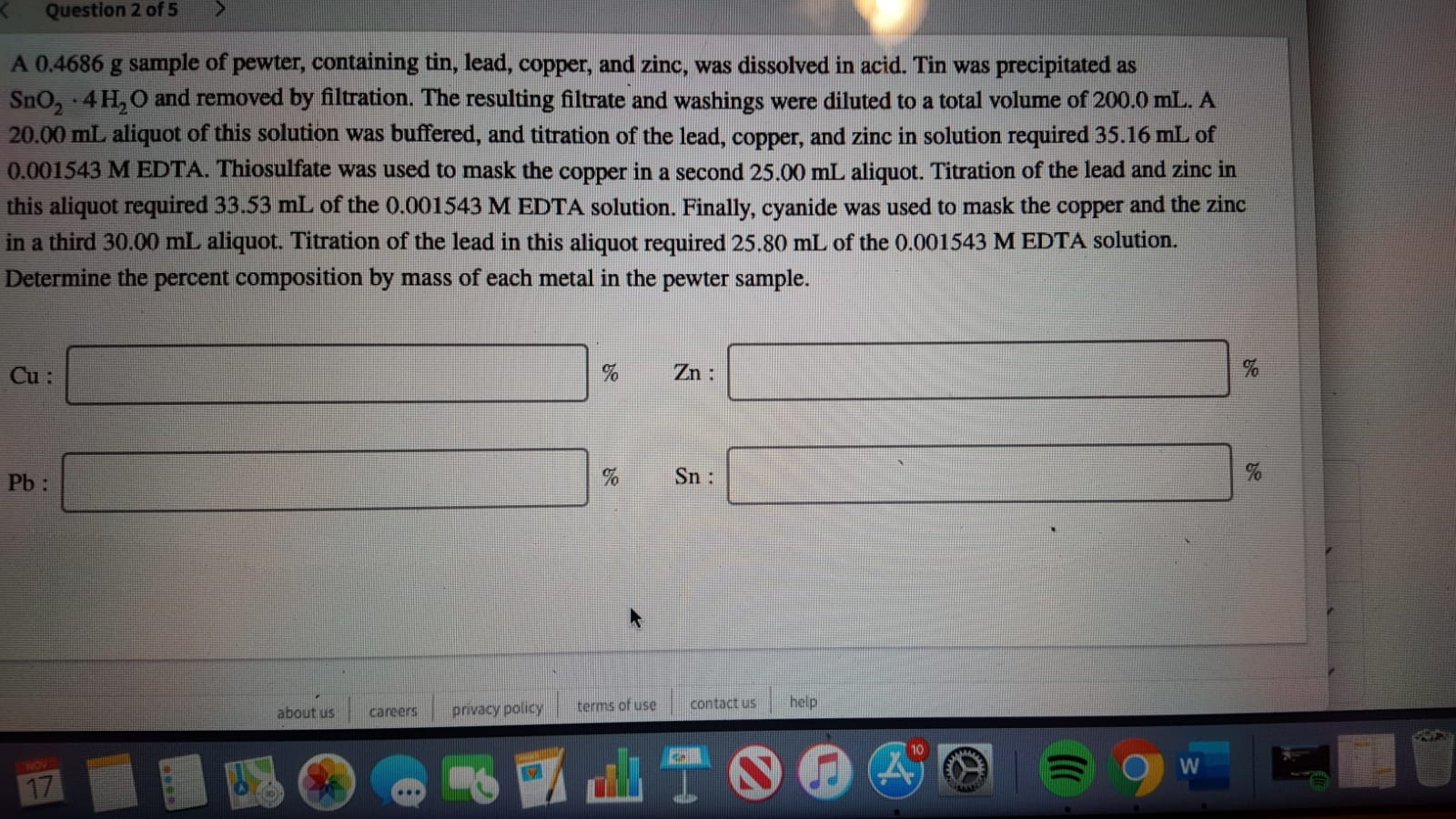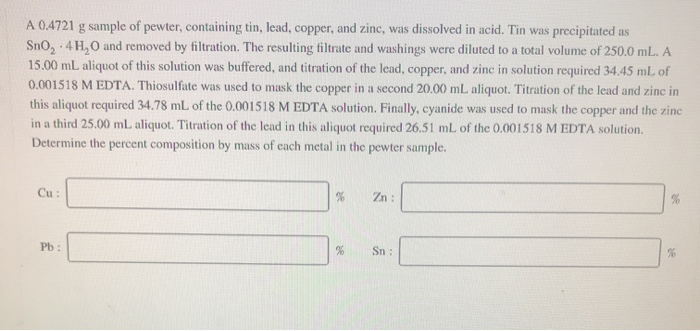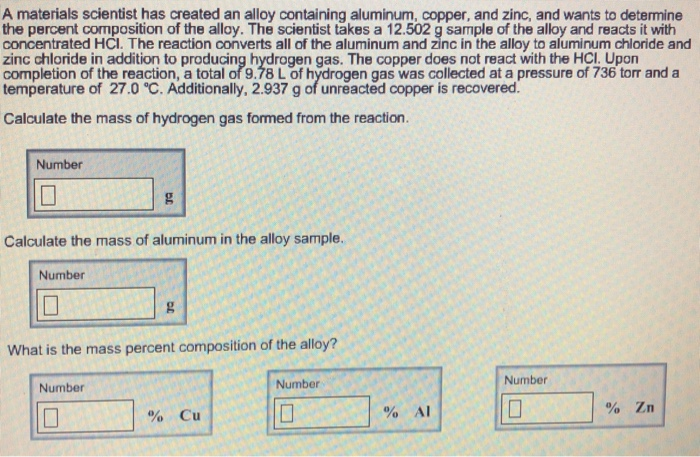Question

# 1. A 0.7336-g sample of an alloy containing copper and zinc is dissolved in 8 M...

1. A 0.7336-g sample of an alloy containing copper and zinc is dissolved in 8 M HCl and diluted to 100 mL in a volumetric flask. In one analysis, the zinc in a 25.00-mL portion of the solution is precipitated as ZnNH4PO4, and subsequently isolated as Zn2P2O7, yielding 0.1163 g. The copper in a separate 25.00-mL portion of the solution is treated to precipitate CuSCN, yielding 0.1931 g. Calculate the %w/w Zn and the %w/w Cu in the sample.

From the recovered Zn2P2O7 we calculate the grams of Zn recovered:

g Zn = 0.1163 g Zn2P2O7 * (1 mol Zn2P2O7 / 304.71 g) * (2 mol Zn / 1 mol Zn2P2O7) * (65.38 g Zn / 1 mol) * (100 mL / 25 mL) = 0.2 g Zn recovered

From the precipitate of CuSCN we calculate the grams of Cu recovered:

g Cu = 0.1931 g CuSCN * (1 mole CuSCN / 121.63 g) * (1 mole Cu / 1 mole CuSCN) * (63.55 g Cu / 1 mole) * (100 mL / 25 mL) = 0.4 g Cu recovered

We calculate the percentages by weight of each metal:

% Zn = g Zn * 100 / g total = 0.2 * 100 / 0.7336 = 27.26%

% Cu = 0.4 * 100 / 0.7336 = 54.53%

If you liked the answer, please rate it in a positive way, you would help me a lot, thank you.

#### Earn Coins

Coins can be redeemed for fabulous gifts.

Similar Homework Help Questions
• ### A 0.4505 g sample of pewter, containing tin, lead, copper, and zinc, was dissolved in acid....

A 0.4505 g sample of pewter, containing tin, lead, copper, and zinc, was dissolved in acid. Tin was precipitated as SnO2⋅4H2Oand removed by filtration. The resulting filtrate and washings were diluted to a total volume of 250.0 mL. A 15.00 mL aliquot of this solution was buffered, and titration of the lead, copper, and zinc in solution required 35.10 mL of 0.001497 M EDTA. Thiosulfate was used to mask the copper in a second 20.00 mL aliquot. Titration of the...

• ### A 0.4598 g sample of pewter, containing tin, lead, copper, and zinc, was dissolved in acid....

A 0.4598 g sample of pewter, containing tin, lead, copper, and zinc, was dissolved in acid. Tin was precipitated as SnO2·4H2O and removed by filtration. The resulting filtrate and washings were diluted to a total volume of 250.0 mL. A 20.00 mL aliquot of this solution was buffered, and titration of the lead, copper, and zinc in solution required 34.81 mL of 0.001487 M EDTA. Thiosulfate was used to mask the copper in a second 25.00 mL aliquot. Titration of...

• ### A 0.4504 g sample of pewter, containing tin, lead, copper, and zinc, was dissolved in acid....

A 0.4504 g sample of pewter, containing tin, lead, copper, and zinc, was dissolved in acid. Tin was precipitated as SnO2⋅4H2O and removed by filtration. The resulting filtrate and washings were diluted to a total volume of 250.0 mL. A 15.00 mL aliquot of this solution was buffered, and titration of the lead, copper, and zinc in solution required 35.78 mL of 0.001478 M EDTA. Thiosulfate was used to mask the copper in a second 20.00 mL aliquot. Titration of...

• ### A 0.3304-g sample of brass (containing lead, zinc, copper, and tin) was dissolved in nitric acid....

A 0.3304-g sample of brass (containing lead, zinc, copper, and tin) was dissolved in nitric acid. The sparingly soluble SnO2.4H2O was removed by filtration, and the combined filtrate and washings were then diluted to 500.0 mL. A 10.00-mL aliquot was suitably buffered; titration of the lead, zinc, and copper in this aliquot required 34.78 mL of 0.002700 M EDTA. The copper in a 25.00-mL aliquot was masked with thiosulfate; the lead and zinc were then titrated with 25.62 mL of...

• ### Question 2 of 5 A 0.4686 g sample of pewter, containing tin, lead, copper, and zinc,...Question 2 of 5 A 0.4686 g sample of pewter, containing tin, lead, copper, and zinc, was dissolved in acid. Tin was precipitated as Sno, .4H, O and removed by filtration. The resulting filtrate and washings were diluted to a total volume of 200.0 mL. A 20.00 mL aliquot of this solution was buffered, and titration of the lead, copper, and zinc in solution required 35.16 mL of 0.001543 M EDTA. Thiosulfate was used to mask the copper in a...

• ### A 0.6004 g sample of Ni/Cu condenser tubing was dissolved in acid and diluted to 100.0...

A 0.6004 g sample of Ni/Cu condenser tubing was dissolved in acid and diluted to 100.0 mL via a volumetric flask. Titration of both cations in a 25.00 mL aliquot of this solution required 45.81 mL of 0.05285 M EDTA. Mercaptoacetic acid and NH3 were then introduced; production of the Cu complex with the former resulted in the release of an equivalent amount of EDTA, which required a 22.85 mL titration with 0.07238 M Mg2+. Calculate the percentages of Cu...Please help. I will rate! A 0.4721 g sample of pewter, containing tin, lead, copper, and zinc, was dissolved in acid. Tin was precipitated as SnO 4 H,O and removed by filtration. The resulting filtrate and washings were diluted to a total volume of 250.0 mL. A 15.00 mL aliquot of this solution was buffered, and titration of the lead, copper, and zinc in solution required 34.45 mL of 0.001518 M EDTA. Thiosulfate was used to mask the copper in...

• ### A materials scientist has created an alloy containing aluminum, copper, and zinc, and wants to determine the percent composition of the alloy. The scientist takes a 12.502 g sample of the alloy a...A materials scientist has created an alloy containing aluminum, copper, and zinc, and wants to determine the percent composition of the alloy. The scientist takes a 12.502 g sample of the alloy and reacts it with concentrated HCI. The reaction converts all of the aluminum and zinc in the alloy to aluminum chloride and zinc chloride in addition to producing hydrogen gas. The copper does not react with the HCI. Upon completion of the reaction, a total of 9.78 L...

• ### A penny having a mass of 2.5340 g was dissolved in 20 mL of 8 M HNO3. The resulting solution was transferred to a 100.00-ml volumetric flask and diluted to the mark with water.

A penny having a mass of 2.5340 g was dissolved in 20 mL of 8 M HNO3. The resulting solution was transferred to a 100.00-ml volumetric flask and diluted to the mark with water. Then, a sample solution was prepared by transferring 10.00 mL of this solution to a 25.00- ml volumetric flask, adding 2.00 mL of 15 M NH3 and diluting to the mark with waterThe concentration of [Cu(NH3)4]2+ in the sample solution (determined using a Spectro Vis spectrophotometer)...

• ### A tablet weighing 2.074 g and containing aluminium salts was dissolved and diluted to 100.0 mL...

A tablet weighing 2.074 g and containing aluminium salts was dissolved and diluted to 100.0 mL in a volumetric flask. A 25.00 mL aliquot was removed and treated with enough ammonia to precipitate the aluminium (Al) present as aluminium hydroxide (Al(OH)3). After ignition at 800 oC, this precipitate was converted to aluminium oxide (Al2O3) weighed 0.1967 g. Calculate the Al% in the tablet.